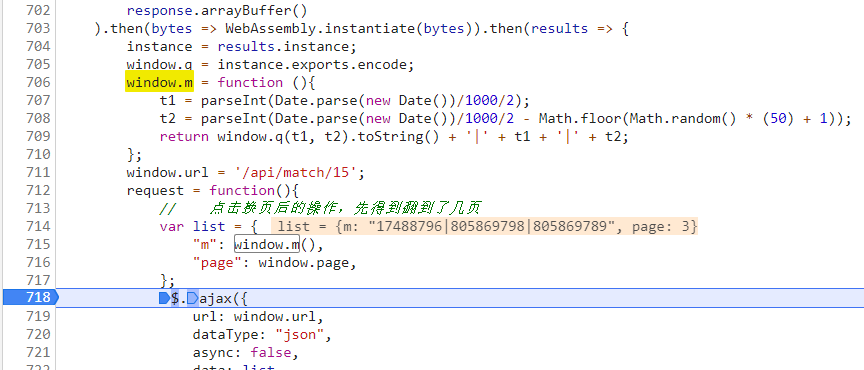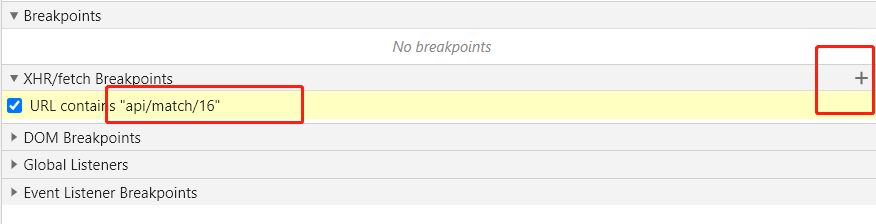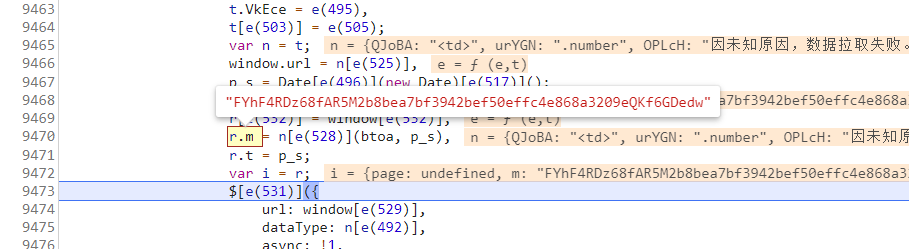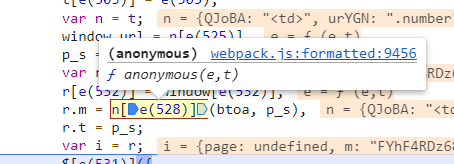出租广告位,需要合作请联系站长

+关注

2020-07(4)

2020-08(27)

## js逆向-猿人学(15-16)wasm-webpack

### 猿人学爬虫第十五题《备周则意怠-常见则不疑》``````fetch('/static/match/match15/main.wasm').then(response =>
response.arrayBuffer()
).then(bytes => WebAssembly.instantiate(bytes)).then(results)
``````

python调用wasm的库： https://www.cnpython.com/pypi/pywasm

instance = results.instance;
window.q = instance.exports.encode;

``````import math
import random,time
import pywasm

t = int(time.time())
t1 = int(t / 2)
t2 = int(t / 2 - math.floor(random.random() * 50 + 1))
sign = wasm.exec("encode", [t1, t2])
m = f"{sign}|{t1}|{t2}"
``````

### 猿人学爬虫第十六题《`webpack`》

webpack之前遇到过很多次了，对于打了包的js，调试十分难受。call stack 往下找 m参数m = n[e(528)](btoa, p_s),

(btoa, p_s) 时间戳，先不看。

n[e(528)] = function(e, t)7 0

pdf(new) 更多>